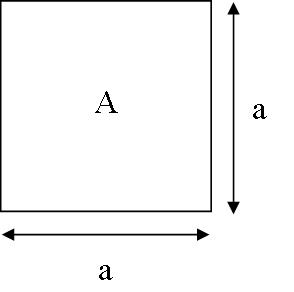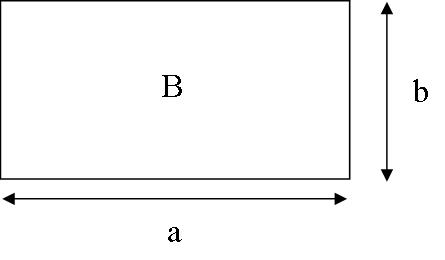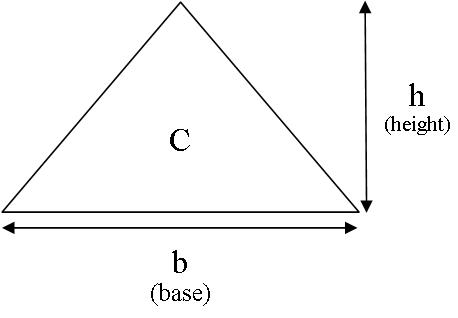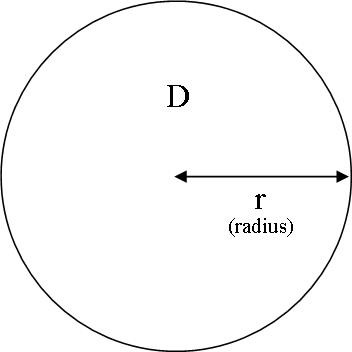01 Oct 2014

# How to calculate areas, part 1

#### Knowing how to calculate areas is an important task when planning your DIY or building project.

You might want to know a given area for many reasons. For instance,

• You want to know the surface area of a wall to calculate the amount of cedar shiplap or feather edge you will need.
• You want to calculate the area of a patio you want to install to calucate the quantity of decking or paving slabs that you require.
• You want to know the area of your roof to calculate how many cedar shingle or slates that you need..

Calculating area is an easy task when you know the right formulas. Most shapes used in building and construction are based on squares, rectangle, triangle and circles. The formulas for these shapes are shown below.

These formulas will help you how to calculate basic areas for your DIY and building projects. This will make sure that you order the correct quantity of building materials to ensure that your job does not come grinding to halt because you have not ordered enough.

#### Area of a squareArea A = a x a = a2

For example, if the sides of the square, a, are 3.0m the area of the square is,

3.0 x 3.0 = 9.0 m2

####Area B =  a x b

For example, if the sides a is 3.0m and side b is 2.0m then the area of the rectangle is,

3.0 x 2.0 = 6.0 m2

#### Area of a triangleArea C =  ½ (b x h)

For example, if the base, b, is 3.0m and the height, h, is 2.5m then the area of the triangle is,

½ (3.0 x 2.5) = 3.75 m2

#### Area of a circle

We need to use the irrational number pi, Π, to calculate the area of a circle.

Don’t worry too much about the value of pi. Use 3.142 which will be accurate enough for most calculations for area. If you need greater accuracy than this you will know this and how to obtain a more accurate value for pi.Area D =  Π x r2

For example, if the radius, is 3.0m then the area of the circle is approximately,

3.142 x (3.0)2 = 28.3 m (to 1 d.p.)